Next: Sampling from Near-identical Distributions Up: Convergence Theorems in Generalized Previous: The Convergence of a

## The Convergence of the Generalized Q-learning Algorithm

It is an easy consequence of Theorem A.1 that under appropriate conditions, the Q-learning algorithm is convergent.

Theorem A.3   If Assumption A holds, and the learning rates satisfy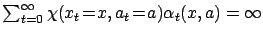and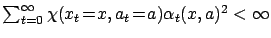uniformly w.p.1, then the generalized Q-learning algorithm described by iteration (Equation 3) converges to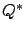w.p.1 uniformly over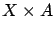.

Proof. The theorem is an easy consequence of Theorem A.1 with the appropriate substitutions and assignments:

Consider the substitution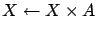,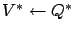and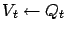. Furthermore, let the randomized approximate dynamic programming operator sequence defined by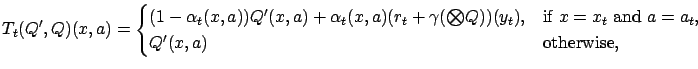which approximates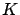atw.p.1 overunder the assumptions (2)-(4) by the well-known Robbins-Monro theorem [Robbins and Monro(1951)].

Finally, let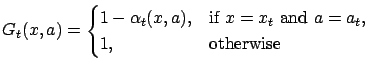(11)

and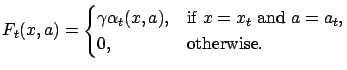(12)

Condition (4) of Theorem A.1 trivially holds, while conditions (1) and (2) are implied by the definition of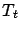and the non-expansion property of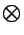. Finally, condition (3) is a consequence of assumption (3) and the definition of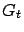.

Applying Theorem A.1 proves the statement.Next: Sampling from Near-identical Distributions Up: Convergence Theorems in Generalized Previous: The Convergence of a